# Fiducial distribution

(diff) ← Older revision | Latest revision (diff) | Newer revision → (diff)
A distributionof the parameterfrom a family of distributionsof an observation. It was introduced by R.A. Fisher  for numericalandin the case when the distribution functionofdecreases asincreases in such a way that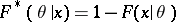, considered as a function offor fixed, has the properties of a distribution function (in such a situation one often makes use of a sufficient statistic in the role of).
A fiducial distribution is defined for invariant families of distributions (cf. ). Namely, suppose that a groupof transformationsacts on the setsand. A family of distributions is called invariant if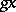has the distribution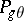whenhas the distribution. In this case one considers equivariant decision rules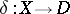(i.e. such that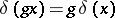for alland) and invariant loss functions(i.e. such that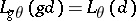for all,and). If the action ofonis transitive, then the family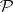has a certain property of homogeneity: For a fixed parameter value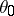and an observationwith the distribution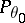, the distribution ofruns through the whole familyasruns through. Suppose thatis a set of probability measures on(it is assumed that-algebras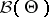andare given such that the transformations inare measurable). Let the action ofonbe given by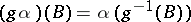,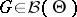. The fiducial distribution is described by the family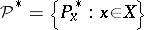of probability measures onthat minimize the risk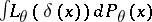in the class of equivariant decision rules for every invariant loss function satisfying the following condition of unbiasedness type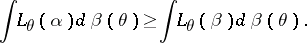Ifacts transitively on, then the family of the fiducial distributions is uniquely distinguished by the requirements thatis invariant and that the probable and fiducial probabilities are equal,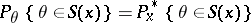, for invariant families(is called invariant if,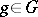imply that).